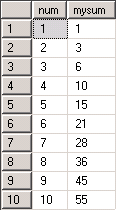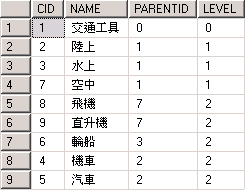# 使用CTE進行遞迴(Recursive)查詢

```WITH cte (num, mysum) AS (
SELECT 1 as num, 1 as mysum
UNION ALL
SELECT num + 1, mysum + num + 1 FROM cte a where num <10
)
SELECT * FROM cte
```是的, 結果就是那個 1 + 2 + 3 + … + 10 = 55 .

```CREATE TABLE CATEGORY (CID int primary key, NAME nvarchar(200), PARENTID int)
INSERT INTO CATEGORY values (1, N'交通工具', 0), (2, N'陸上', 1), (3, N'水上', 1)
INSERT INTO CATEGORY values (4, N'機車', 2), (5, N'汽車', 2), (6, N'輪船', 3)
INSERT INTO CATEGORY values (7, N'空中', 1), (8, N'飛機', 7), (9, N'直升機', 7)
```

```WITH CTE (CID, NAME, PARENTID, LEVEL) AS (
SELECT CID, NAME, PARENTID, 0 FROM CATEGORY WHERE PARENTID = 0
UNION ALL
SELECT A.CID, A.NAME, A.PARENTID, LEVEL + 1 FROM CATEGORY A INNER JOIN CTE B ON A.PARENTID = B.CID
)
SELECT * FROM CTE``````WITH CTE (CID, NAME, PARENTID, LEVEL, ORICID, DATA) AS (
SELECT CID, NAME, PARENTID, 0, CID, CONVERT(NVARCHAR(MAX), NAME) FROM CATEGORY WHERE PARENTID = 0
UNION ALL
SELECT A.CID, A.NAME, A.PARENTID, LEVEL + 1, A.CID, DATA + '-' + A.NAME FROM CATEGORY A INNER JOIN CTE B ON A.PARENTID = B.CID
)
SELECT * FROM CTE
```Types don’t match between the anchor and the recursive part in column “xx” of recursive query “xx”.